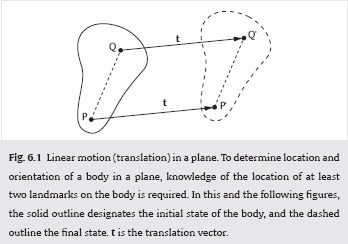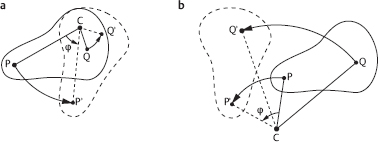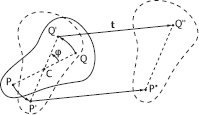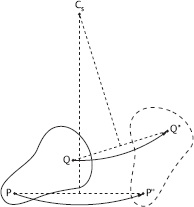# 6 Translation and Rotation in a Plane

10.1055/b-0036-140421

## 6 Translation and Rotation in a Plane

Describing body movements is a frequently occurring problem in orthopedic biomechanics. An example of such a motion is the forward bending of the trunk to grasp an object from the floor. At the beginning, the trunk is erect (initial state); at the end it is bent forward (final state). Other examples of movements are the relative motion of adjacent vertebrae while bending forward or the motion of the lower leg in relation to the upper leg when walking. The description of position and orientation is a geometrical problem, and beyond the simple level such problems are solved algebraically. Indeed, for measurements and calculations, especially in three dimensions, algebraic methods must be used. Initially, however, algebra can be dispensed with by analyzing the motion occurring in a plane graphically, using a pair of compasses and a ruler. This is the content of this chapter.

### 6.1 Graphical Description of Translation and Rotation

In two dimensions we are dealing with plane objects moving in a plane. It is assumed that the objects are rigid (that is, not deformable). This means that their shape and size (internal distances, angles) does not change under the influence of forces. The assumptions of bodies being rigid and movement being confined to a plane are model assumptions frequently used in orthopedic biomechanics. For example, the motion of the lower leg and thigh in walking can be described in the sagittal plane with these two assumptions in mind. In reality, however, the lower leg and thigh are not rigid bodies like the limbs of a puppet; they change their shape noticeably when moving.

To describe the motion of a rigid body in a plane it is not necessary to describe the motion of all points of the body; it is sufficient to describe the motion of two specific points on the body. In what follows, these points will be termed landmarks. This term is used because, in many practical applications, markers are affixed to the bodies to be observed. The markers are constructed so as to be clearly visible in film, video, or radiographic images. If two markers are fixed on a rigid body, a reference system is created. The location of all points of the body can be unequivocally specified in relation to this reference system. It follows that, if the location of the landmarks is known, the location of all other points of the body is known as well. For this reason, the description of plane motion of a rigid body may be confined to describing the motion of two landmarks.

#### 6.1.1 Translation

A motion that moves all points of a body on straight lines over identical distances is termed linear movement or translation. In Fig. 6.1 two markers P and Q are fixed to a body. The body is moved along a straight line. At the end of the motion the location of the markers is designated by Pʹ and Qʹ. Since, by definition, all points of the body have been moved along straight lines and over identical distances, the lines Pʹ and QQʹ are parallel and of equal length. Since the body was assumed to be rigid (that is, not deformable), the lines PQ and PʹQʹ are of equal length as well. It follows that the points P, Pʹ, Qʹ, Q form the corners of a parallelogram. In other words, if the initial and the final state of the motion are known, and if the points P, Pʹ, Qʹ, Q (in that order) form the corners of a parallelogram, the movement can be interpreted as a translation. Fig. 6.1 shows the identical vectors PPʹ = t and QQʹ = t. The magnitude and direction of t characterize the translation.Fig. 6.1 Linear motion (translation) in a plane. To determine location and orientation of a body in a plane, knowledge of the location of at least two landmarks on the body is required. In this and the following figures, the solid outline designates the initial state of the body, and the dashed outline the final state. t is the translation vector.

#### 6.1.2 Rotation

A rotation is characterized by the fact that all points of a body move on concentric circles with the identical angle of rotation around a center of rotation. In Fig. 6.2 the landmarks P and Q move along arcs of circles to Pʹ and Qʹ. In Fig. 6.2a the center of rotation C is located within the boundary and in Fig. 6.2b it is located outside the boundary of the rotating body. In both cases the angle between the lines PQ and PʹQʹ equalsφ. The rotation has a direction; accordingly the angle of rotation may be positive or negative. A positive angle characterizes a counterclockwise rotation, a negative angle characterizes a clockwise rotation. A plane rotation is unequivocally specified by the location of the center of rotation and the angle of rotation.Fig. 6.2a, b Rotation in a plane. All points are rotated about the identical center of rotation C by the angle of rotation φ; the points move on arcs of concentric circles around the center of rotation. a The center of rotation is located within the boundary of the body. b The center of rotation is located outside the boundary of the body.

If the initial and final states of the body are known, the position of the center of rotation and the angle of rotation may be reconstructed ( Fig. 6.3 ). Points P and Pʹ and points Q and Qʹ lie on arcs of circles around the (as yet unknown) center of rotation C. If lines PPʹ and QQʹ are bisected perpendicularly, the center of rotation C is located at the intersection of these perpendicular bisectors ( Fig. 6.3a ). The assumption underlying this construction is that the perpendicular bisectors are differently orientated (they are not parallel). In the special case when they are orientated identically (they are parallel) ( Fig. 6.3b ), points P, Q, and the center of rotation C lie on a straight line. In this case the center of rotation C is given by the intersection of the lines PQ and PʹQʹ. In both cases the angle of rotation is given by the angle between the lines PQ and PʹQʹ.Fig. 6.3a, b Construction of the location of the center of rotation of a plane rotation. a Standard case (nonparallel bisectors). b Special case (parallel bisectors).

#### 6.1.3 Combined Translation and Rotation

In general, a motion may combine translation and rotation. In the example given in Fig. 6.4 the body is rotated in a first step around C by an angle φ in counterclockwise direction. Through this rotation, points P and Q are moved on arcs of circles to Pʹ and Qʹ. In a second step the body is moved along a straight line. The final locations of the landmarks are Pʹʹ and Qʹʹ. The translation is characterized by the translation vector t = QʹQʹʹ.Fig. 6.4 Plane motion combining rotation and translation. In a first step the body is rotated through an angle φ around the center of rotation C; in a second step the body is translated as indicated by the translation vector t.

If the initial and final state of the motion are known, the angle of rotation φ can be obtained by rotating the body in the final state around an arbitrarily chosen center of rotation until the lines connecting the landmarks are parallel. In a second step a translation may be performed until the landmarks coincide. The translation vector thus obtained depends on the preceding choice of the location of the center of rotation; in general it is not identical with t. In other words: when describing a motion that combines rotation and translation, only the angle of rotation is unequivocally determined; the contribution of translational motion depends on the choice of the center of rotation.

For plane motions the following noteworthy theorem holds. Any motion resulting from a combination of translation and rotation can be described by a single rotation. This “substitute” rotation occurs around a center of rotation differing from the original center of rotation but with the identical angle of rotation φ. Fig. 6.5 shows the body in the same initial and final state as in Fig. 6.4 . The construction of the substitute center of rotation Cs is performed using the same rule as illustrated in Fig. 6.3.Fig. 6.5 Location of the substitute center of rotation Cs for the combined motion illustrated in Fig. 6.4 . The location of the substitute center of rotation is constructed according to the rule illustrated in Fig. 6.3a .

The fact that an identical change of location of a body may be described either by a pure rotation or by a combination of translation and rotation emphasizes that the description of a motion provides no information about the actual path of the motion between the initial and the final state. In other words, if only the initial and the final location of a body are known, the actual motion between these locations may have varied considerably. Which description to choose in a given situation—a pure translation or a combination of translation and rotation—depends on the biomechanical model adopted. If, for example, a body is known to move around an axis (for example, the lower leg around the axis of the knee joint), the motion is likely to be described as a pure rotation. If translational or rotational movements are suspected to occur simultaneously or in sequence over time (for example, dorsoventral shift and rotation of a lumbar vertebra when bending forward), one can try to decompose the observed motion into a translational and a rotational part.

#### 6.1.4 Error Influences when Describing a Motion

With small angles of rotation and increasing distance of the center of rotation from the body it becomes increasingly difficult to distinguish a rotation from a translational motion. In Fig. 6.6 the translational motion is depicted by the vector t pointing from P to Pʹt and the rotational motion by the arc b of the circle from P to Pʹr. If the vector t is kept constant and the center of rotation C moves away from P, the radius r of the arc increases and the angle φ decreases. At the same time, arc b tends to coincide with the translation vector t and the distance d between the points Pʹt and Pʹr becomes smaller and smaller. If the center of rotation moves away to infinity, there will be no difference between rotational motion along the arc of the circle and translational motion.Fig. 6.6 Translation as limiting case of a rotation with the center of rotation shifted to infinity. Point P is shifted by the translation vector t to Pʹt.

The very small difference between translation and rotation in a case where the radius is large and the angle of rotation small dictates that caution must be used in interpreting measured data. Because measurement errors can never be avoided, a translation may be wrongly interpreted as a rotation. In general, therefore, it holds that the interpretation of a motion as rotation is reliable only if the center of rotation is located fairly close the body.

Inevitable measurement errors for the coordinates of landmarks, and the difficulty of discriminating between translation and rotation where angles of rotation are small, result in complications if an attempt is made to trace a motion very precisely by dividing its path into many small segments. Let us imagine a body that in reality moves along a smooth curve. Repeated measurement of the body’s location at short intervals splits the path into segments. On average the direction of the segments follows the true curve, but due to measurement errors the direction of individual segments may deviate irregularly from the true (unknown) value. If an attempt were made to describe the motion in each segment of the path by a center and an angle of rotation, a large scatter would probably be found for the location of these centers. Since a zigzag curve of an instantaneous center of rotation cannot be interpreted meaningfully, it is necessary in such cases to smooth the curve mathematically before describing the motion. However, one is now confronted with the problem of selecting a smoothing algorithm (that is, an appropriate mathematical procedure) that leaves the underlying, original curve unchanged.